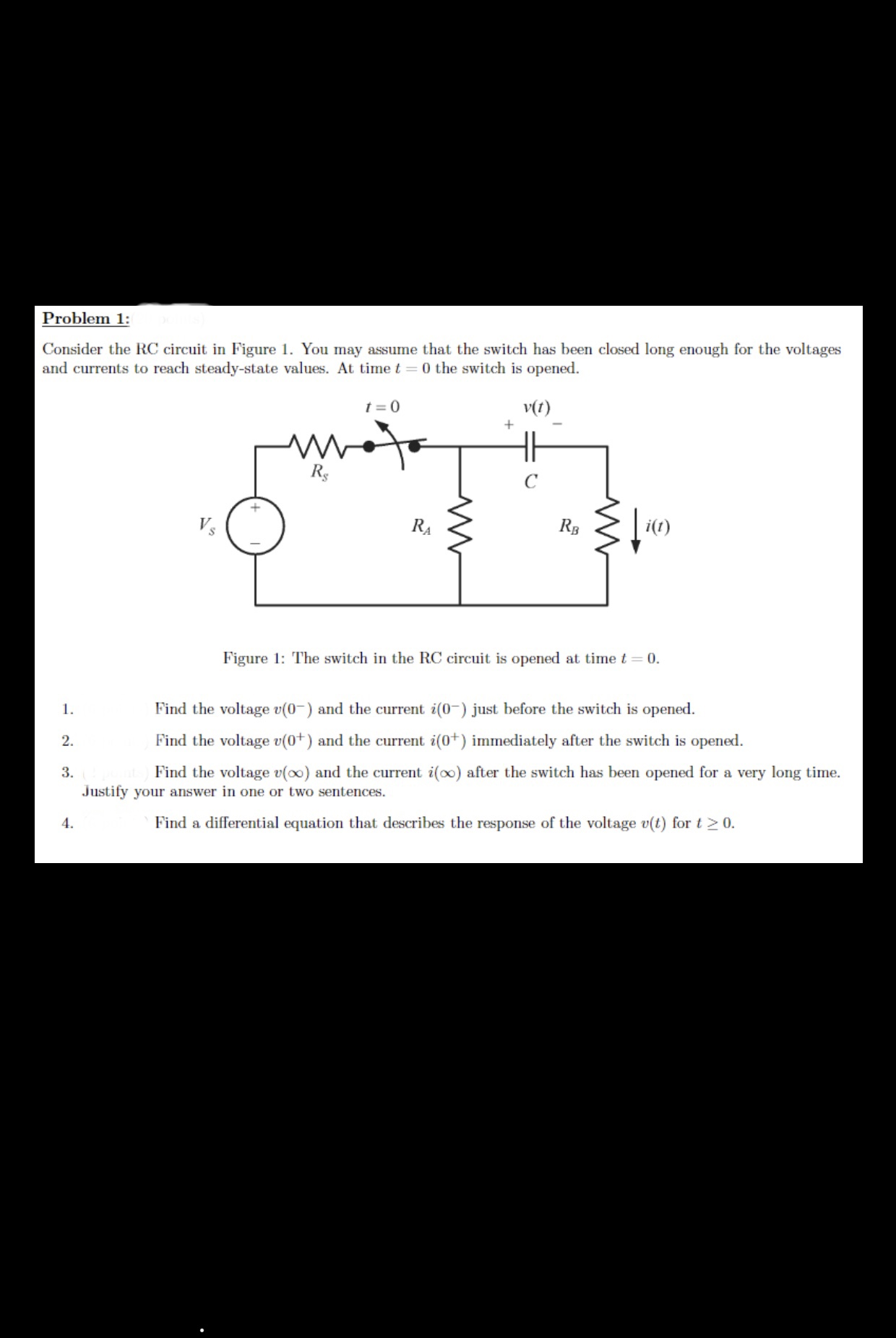# Problem 1:Consider the RC circuit in Figure 1. You may assume that the switch has been closed long enough for the voltagesand currents to reach steady-state values. At time t = 0 the switch is opened0v(t)Rsi(t)VsRBRAFigure 1: The switch in the RC circuit is opened at time t 0Find the voltage v(0and the current i(0just before the switch is opened1.Find the voltage v(0) and the current i(0) immediately after the switch is opened23Justify your answer in one or two sentencesFind the voltage v(oo) and the current i(oo) after the switch has been opened for a very long time.Find a differential equation that describes the response of the voltage v(t) for t 2 0.4

Question
11 viewshelp_outlineImage TranscriptioncloseProblem 1: Consider the RC circuit in Figure 1. You may assume that the switch has been closed long enough for the voltages and currents to reach steady-state values. At time t = 0 the switch is opened 0 v(t) Rs i(t) Vs RB RA Figure 1: The switch in the RC circuit is opened at time t 0 Find the voltage v(0 and the current i(0 just before the switch is opened 1. Find the voltage v(0) and the current i(0) immediately after the switch is opened 2 3 Justify your answer in one or two sentences Find the voltage v(oo) and the current i(oo) after the switch has been opened for a very long time. Find a differential equation that describes the response of the voltage v(t) for t 2 0. 4 fullscreen
check_circle

Step 1

(1)

For the v(0-) and i(0-), the capacitor C acts as open circuit because for a long time, it was closed and it is at steady state. Thus, the current at that instant is,

Step 2

The voltage at that instant is,

Step 3

(2)

When the switch is opened, the capacitor C does not discharge instantane...

### Want to see the full answer?

See Solution

#### Want to see this answer and more?

Solutions are written by subject experts who are available 24/7. Questions are typically answered within 1 hour.*

See Solution
*Response times may vary by subject and question.
Tagged in

### Physics